# The Worksheet Class

The worksheet class represents an Excel worksheet. It handles operations such as writing data to cells or formatting worksheet layout.

A worksheet object isn’t instantiated directly. Instead a new worksheet is created by calling the `add_worksheet()` method from a `Workbook()` object:

```workbook   = xlsxwriter.Workbook('filename.xlsx')

worksheet1.write('A1', 123)

workbook.close()
```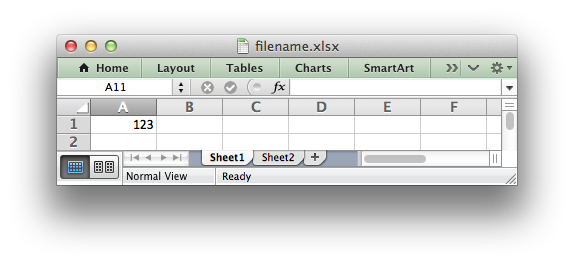XlsxWriter supports Excels worksheet limits of 1,048,576 rows by 16,384 columns.

## worksheet.write()

`write`(row, col, *args)

Write generic data to a worksheet cell.

Parameters: row – The cell row (zero indexed). col – The cell column (zero indexed). *args – The additional args that are passed to the sub methods such as number, string and cell_format. 0: Success. -1: Row or column is out of worksheet bounds. Other values from the called write methods.

Excel makes a distinction between data types such as strings, numbers, blanks, formulas and hyperlinks. To simplify the process of writing data to an XlsxWriter file the `write()` method acts as a general alias for several more specific methods:

The rules for handling data in `write()` are as follows:

Strings are then handled as follows:

• Strings that start with `"="` are assumed to match a formula and are written using `write_formula()`. This can be overridden, see below.
• Strings that match supported URL types are written using `write_url()`. This can be overridden, see below.
• When the `Workbook()` constructor `strings_to_numbers` option is `True` strings that convert to numbers using `float()` are written using `write_number()` in order to avoid Excel warnings about “Numbers Stored as Text”. See the note below.
• Strings that don’t match any of the above criteria are written using `write_string()`.

If none of the above types are matched the value is evaluated with `float()` to see if it corresponds to a user defined float type. If it does then it is written using `write_number()`.

Finally, if none of these rules are matched then a `TypeError` exception is raised. However, it is also possible to handle additional, user defined, data types using the `add_write_handler()` method explained below and in Writing user defined types.

Here are some examples:

```worksheet.write(0, 0, 'Hello')          # write_string()
worksheet.write(1, 0, 'World')          # write_string()
worksheet.write(2, 0, 2)                # write_number()
worksheet.write(3, 0, 3.00001)          # write_number()
worksheet.write(4, 0, '=SIN(PI()/4)')   # write_formula()
worksheet.write(5, 0, '')               # write_blank()
worksheet.write(6, 0, None)             # write_blank()
```

This creates a worksheet like the following:Note

The `Workbook()` constructor option takes three optional arguments that can be used to override string handling in the `write()` function. These options are shown below with their default values:

```xlsxwriter.Workbook(filename, {'strings_to_numbers':  False,
'strings_to_formulas': True,
'strings_to_urls':     True})
```

The `write()` method supports two forms of notation to designate the position of cells: Row-column notation and A1 notation:

```# These are equivalent.
worksheet.write(0, 0, 'Hello')
worksheet.write('A1', 'Hello')
```

See Working with Cell Notation for more details.

The `cell_format` parameter in the sub `write` methods is used to apply formatting to the cell. This parameter is optional but when present it should be a valid Format object:

```cell_format = workbook.add_format({'bold': True, 'italic': True})

worksheet.write(0, 0, 'Hello', cell_format)  # Cell is bold and italic.
```

`add_write_handler`(user_type, user_function)

Add a callback function to the `write()` method to handle user define types.

Parameters: user_type (type) – The user `type()` to match on. user_function (types.FunctionType) – The user defined function to write the type data.

As explained above, the `write()` method maps basic Python types to corresponding Excel types. If you want to write an unsupported type then you can either avoid `write()` and map the user type in your code to one of the more specific write methods or you can extend it using the `add_write_handler()` method.

For example, say you wanted to automatically write `uuid` values as strings using `write()` you would start by creating a function that takes the uuid, converts it to a string and then writes it using `write_string()`:

```def write_uuid(worksheet, row, col, uuid, format=None):
string_uuid = str(uuid)
return worksheet.write_string(row, col, string_uuid, format)
```

You could then add a handler that matches the `uuid` type and calls your user defined function:

```#                           match,     action()
```

Then you can use `write()` without further modification:

```my_uuid = uuid.uuid3(uuid.NAMESPACE_DNS, 'python.org')

# Write the UUID. This would raise a TypeError without the handler.
worksheet.write('A1', my_uuid)
```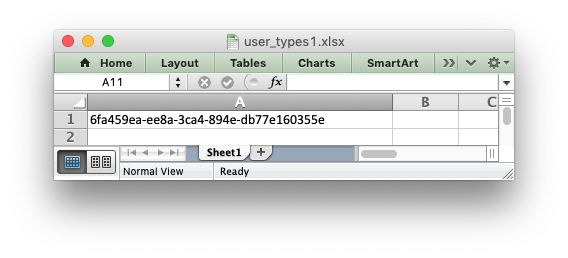Multiple callback functions can be added using `add_write_handler()` but only one callback action is allowed per type. However, it is valid to use the same callback function for different types:

```worksheet.add_write_handler(int,   test_number_range)
```

See Writing user defined types for more details on how this feature works and how to write callback functions, and also the following examples:

## worksheet.write_string()

`write_string`(row, col, string[, cell_format])

Write a string to a worksheet cell.

Parameters: row (int) – The cell row (zero indexed). col (int) – The cell column (zero indexed). string (string) – String to write to cell. cell_format (Format) – Optional Format object. 0: Success. -1: Row or column is out of worksheet bounds. -2: String truncated to 32k characters.

The `write_string()` method writes a string to the cell specified by `row` and `column`:

```worksheet.write_string(0, 0, 'Your text here')
worksheet.write_string('A2', 'or here')
```

Both row-column and A1 style notation are supported, as shown above. See Working with Cell Notation for more details.

The `cell_format` parameter is used to apply formatting to the cell. This parameter is optional but when present is should be a valid Format object.

Unicode strings are supported in UTF-8 encoding. This generally requires that your source file is UTF-8 encoded:

```worksheet.write('A1', u'Some UTF-8 text')
```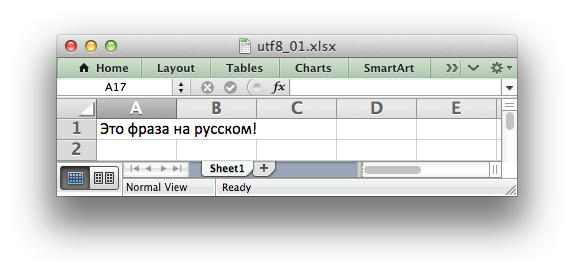See Example: Simple Unicode with Python 3 for a more complete example.

Alternatively, you can read data from an encoded file, convert it to UTF-8 during reading and then write the data to an Excel file. See Example: Unicode - Polish in UTF-8 and Example: Unicode - Shift JIS.

The maximum string size supported by Excel is 32,767 characters. Strings longer than this will be truncated by `write_string()`.

Note

Even though Excel allows strings of 32,767 characters it can only display 1000 in a cell. However, all 32,767 characters are displayed in the formula bar.

## worksheet.write_number()

`write_number`(row, col, number[, cell_format])

Write a number to a worksheet cell.

Parameters: row (int) – The cell row (zero indexed). col (int) – The cell column (zero indexed). number (int or float) – Number to write to cell. cell_format (Format) – Optional Format object. 0: Success. -1: Row or column is out of worksheet bounds.

The `write_number()` method writes numeric types to the cell specified by `row` and `column`:

```worksheet.write_number(0, 0, 123456)
worksheet.write_number('A2', 2.3451)
```

Both row-column and A1 style notation are supported, as shown above. See Working with Cell Notation for more details.

The numeric types supported are `float`, `int`, `long`, `decimal.Decimal` and `fractions.Fraction` or anything that can be converted via `float()`.

When written to an Excel file numbers are converted to IEEE-754 64-bit double-precision floating point. This means that, in most cases, the maximum number of digits that can be stored in Excel without losing precision is 15.

Note

NAN and INF are not supported and will raise a TypeError exception unless the `nan_inf_to_errors` Workbook() option is used.

The `cell_format` parameter is used to apply formatting to the cell. This parameter is optional but when present is should be a valid Format object.

## worksheet.write_formula()

`write_formula`(row, col, formula[, cell_format[, value]])

Write a formula to a worksheet cell.

Parameters: row (int) – The cell row (zero indexed). col (int) – The cell column (zero indexed). formula (string) – Formula to write to cell. cell_format (Format) – Optional Format object. value – Optional result. The value if the formula was calculated. 0: Success. -1: Row or column is out of worksheet bounds.

The `write_formula()` method writes a formula or function to the cell specified by `row` and `column`:

```worksheet.write_formula(0, 0, '=B3 + B4')
worksheet.write_formula(1, 0, '=SIN(PI()/4)')
worksheet.write_formula(2, 0, '=SUM(B1:B5)')
worksheet.write_formula('A4', '=IF(A3>1,"Yes", "No")')
worksheet.write_formula('A5', '=AVERAGE(1, 2, 3, 4)')
worksheet.write_formula('A6', '=DATEVALUE("1-Jan-2013")')
```

Both row-column and A1 style notation are supported, as shown above. See Working with Cell Notation for more details.

Array formulas are also supported:

```worksheet.write_formula('A7', '{=SUM(A1:B1*A2:B2)}')
```

See also the `write_array_formula()` method below.

The `cell_format` parameter is used to apply formatting to the cell. This parameter is optional but when present is should be a valid Format object.

If required, it is also possible to specify the calculated result of the formula using the optional `value` parameter. This is occasionally necessary when working with non-Excel applications that don’t calculate the result of the formula:

```worksheet.write('A1', '=2+2', num_format, 4)
```

See Formula Results for more details.

Excel stores formulas in US style formatting regardless of the Locale or Language of the Excel version:

```worksheet.write_formula('A1', '=SUM(1, 2, 3)')    # OK
worksheet.write_formula('A2', '=SOMME(1, 2, 3)')  # French. Error on load.
```

See Non US Excel functions and syntax for a full explanation.

Excel 2010 and 2013 added functions which weren’t defined in the original file specification. These functions are referred to as future functions. Examples of these functions are `ACOT`, `CHISQ.DIST.RT` , `CONFIDENCE.NORM`, `STDEV.P`, `STDEV.S` and `WORKDAY.INTL`. In XlsxWriter these require a prefix:

```worksheet.write_formula('A1', '=_xlfn.STDEV.S(B1:B10)')
```

See Formulas added in Excel 2010 and later for a detailed explanation and full list of functions that are affected.

## worksheet.write_array_formula()

`write_array_formula`(first_row, first_col, last_row, last_col, formula[, cell_format[, value]])

Write an array formula to a worksheet cell.

Parameters: first_row (int) – The first row of the range. (All zero indexed.) first_col (int) – The first column of the range. last_row (int) – The last row of the range. last_col (int) – The last col of the range. formula (string) – Array formula to write to cell. cell_format (Format) – Optional Format object. value – Optional result. The value if the formula was calculated. 0: Success. -1: Row or column is out of worksheet bounds.

The `write_array_formula()` method writes an array formula to a cell range. In Excel an array formula is a formula that performs a calculation on a set of values. It can return a single value or a range of values.

An array formula is indicated by a pair of braces around the formula: `{=SUM(A1:B1*A2:B2)}`.

For array formulas that return a range of values you must specify the range that the return values will be written to:

```worksheet.write_array_formula(0, 0, 2, 0, '{=TREND(C1:C3,B1:B3)}')
worksheet.write_array_formula('A1:A3',    '{=TREND(C1:C3,B1:B3)}')
```

Both row-column and A1 style notation are supported, as shown above. See Working with Cell Notation for more details.

If the array formula returns a single value then the `first_` and `last_` parameters should be the same:

```worksheet.write_array_formula('A1:A1', '{=SUM(B1:C1*B2:C2)}')
```

It this case however it is easier to just use the `write_formula()` or `write()` methods:

```# Same as above but more concise.
worksheet.write('A1', '{=SUM(B1:C1*B2:C2)}')
worksheet.write_formula('A1', '{=SUM(B1:C1*B2:C2)}')
```

The `cell_format` parameter is used to apply formatting to the cell. This parameter is optional but when present is should be a valid Format object.

If required, it is also possible to specify the calculated result of the formula (see discussion of formulas and the `value` parameter for the `write_formula()` method above). However, using this parameter only writes a single value to the upper left cell in the result array. See Formula Results for more details.

## worksheet.write_dynamic_array_formula()

`write_dynamic_array_formula`(first_row, first_col, last_row, last_col, formula[, cell_format[, value]])

Write an array formula to a worksheet cell.

Parameters: first_row (int) – The first row of the range. (All zero indexed.) first_col (int) – The first column of the range. last_row (int) – The last row of the range. last_col (int) – The last col of the range. formula (string) – Array formula to write to cell. cell_format (Format) – Optional Format object. value – Optional result. The value if the formula was calculated. 0: Success. -1: Row or column is out of worksheet bounds.

The `write_dynamic_array_formula()` method writes an dynamic array formula to a cell range. Dynamic array formulas are explained in detail in Dynamic Array support.

The syntax of `write_dynamic_array_formula()` is the same as `write_array_formula()`, shown above, except that you don’t need to add `{}` braces:

```worksheet.write_dynamic_array_formula('B1:B3', '=LEN(A1:A3)')
```

Which gives the following result: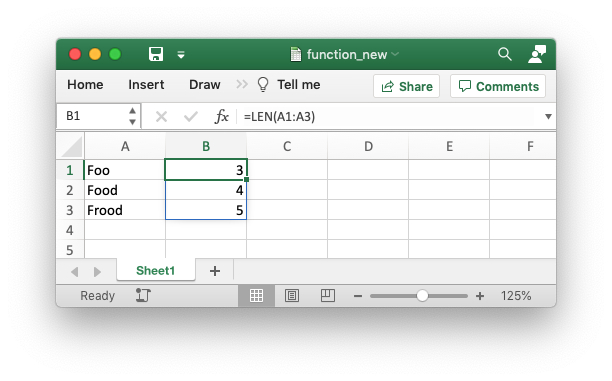It is also possible to specify the first cell of the range to get the same results:

```worksheet.write_dynamic_array_formula('B1:B1', '=LEN(A1:A3)')
```

## worksheet.write_blank()

`write_blank`(row, col, blank[, cell_format])

Write a blank worksheet cell.

Parameters: row (int) – The cell row (zero indexed). col (int) – The cell column (zero indexed). blank – None or empty string. The value is ignored. cell_format (Format) – Optional Format object. 0: Success. -1: Row or column is out of worksheet bounds.

Write a blank cell specified by `row` and `column`:

```worksheet.write_blank(0, 0, None, cell_format)
worksheet.write_blank('A2', None, cell_format)
```

Both row-column and A1 style notation are supported, as shown above. See Working with Cell Notation for more details.

This method is used to add formatting to a cell which doesn’t contain a string or number value.

Excel differentiates between an “Empty” cell and a “Blank” cell. An “Empty” cell is a cell which doesn’t contain data or formatting whilst a “Blank” cell doesn’t contain data but does contain formatting. Excel stores “Blank” cells but ignores “Empty” cells.

As such, if you write an empty cell without formatting it is ignored:

```worksheet.write('A1', None, cell_format)  # write_blank()
worksheet.write('A2', None)               # Ignored
```

This seemingly uninteresting fact means that you can write arrays of data without special treatment for `None` or empty string values.

## worksheet.write_boolean()

`write_boolean`(row, col, boolean[, cell_format])

Write a boolean value to a worksheet cell.

Parameters: row (int) – The cell row (zero indexed). col (int) – The cell column (zero indexed). boolean (bool) – Boolean value to write to cell. cell_format (Format) – Optional Format object. 0: Success. -1: Row or column is out of worksheet bounds.

The `write_boolean()` method writes a boolean value to the cell specified by `row` and `column`:

```worksheet.write_boolean(0, 0, True)
worksheet.write_boolean('A2', False)
```

Both row-column and A1 style notation are supported, as shown above. See Working with Cell Notation for more details.

The `cell_format` parameter is used to apply formatting to the cell. This parameter is optional but when present is should be a valid Format object.

## worksheet.write_datetime()

`write_datetime`(row, col, datetime[, cell_format])

Write a date or time to a worksheet cell.

Parameters: row (int) – The cell row (zero indexed). col (int) – The cell column (zero indexed). datetime (`datetime`) – A datetime.datetime, .date, .time or .delta object. cell_format (Format) – Optional Format object. 0: Success. -1: Row or column is out of worksheet bounds.

The `write_datetime()` method can be used to write a date or time to the cell specified by `row` and `column`:

```worksheet.write_datetime(0, 0, datetime, date_format)
worksheet.write_datetime('A2', datetime, date_format)
```

Both row-column and A1 style notation are supported, as shown above. See Working with Cell Notation for more details.

The datetime should be a `datetime.datetime`, `datetime.date` `datetime.time` or `datetime.timedelta` object. The `datetime` class is part of the standard Python libraries.

There are many ways to create datetime objects, for example the `datetime.datetime.strptime()` method:

```date_time = datetime.datetime.strptime('2013-01-23', '%Y-%m-%d')
```

See the `datetime` documentation for other date/time creation methods.

A date/time should have a `cell_format` of type Format, otherwise it will appear as a number:

```date_format = workbook.add_format({'num_format': 'd mmmm yyyy'})

worksheet.write_datetime('A1', date_time, date_format)
```

If required, a default date format string can be set using the `Workbook()` constructor `default_date_format` option.

See Working with Dates and Time for more details and also Timezone Handling in XlsxWriter.

## worksheet.write_url()

`write_url`(row, col, url[, cell_format[, string[, tip]]])

Write a hyperlink to a worksheet cell.

Parameters: row (int) – The cell row (zero indexed). col (int) – The cell column (zero indexed). url (string) – Hyperlink url. cell_format (Format) – Optional Format object. Defaults to the Excel hyperlink style. string (string) – An optional display string for the hyperlink. tip (string) – An optional tooltip. 0: Success. -1: Row or column is out of worksheet bounds. -2: String longer than 32k characters. -3: Url longer than Excel limit of 2079 characters. -4: Exceeds Excel limit of 65,530 urls per worksheet.

The `write_url()` method is used to write a hyperlink in a worksheet cell. The url is comprised of two elements: the displayed string and the non-displayed link. The displayed string is the same as the link unless an alternative string is specified:

```worksheet.write_url(0, 0, 'https://www.python.org/')
worksheet.write_url('A2', 'https://www.python.org/')
```

Both row-column and A1 style notation are supported, as shown above. See Working with Cell Notation for more details.

The `cell_format` parameter is used to apply formatting to the cell. This parameter is optional and the default Excel hyperlink style will be used if it isn’t specified. If required you can access the default url format using the Workbook `get_default_url_format()` method:

```url_format = workbook.get_default_url_format()
```

Four web style URI’s are supported: `http://`, `https://`, `ftp://` and `mailto:`:

```worksheet.write_url('A1', 'ftp://www.python.org/')
worksheet.write_url('A2', 'https://www.python.org/')
worksheet.write_url('A3', 'mailto:jmcnamara@cpan.org')
```

All of the these URI types are recognized by the `write()` method, so the following are equivalent:

```worksheet.write_url('A2', 'https://www.python.org/')
worksheet.write    ('A2', 'https://www.python.org/')  # Same.
```

You can display an alternative string using the `string` parameter:

```worksheet.write_url('A1', 'https://www.python.org', string='Python home')
```

Note

If you wish to have some other cell data such as a number or a formula you can overwrite the cell using another call to `write_*()`:

```worksheet.write_url('A1', 'https://www.python.org/')

# Overwrite the URL string with a formula. The cell will still be a link.
# Note the use of the default url format for consistency with other links.
url_format = workbook.get_default_url_format()
worksheet.write_formula('A1', '=1+1', url_format)
```

There are two local URIs supported: `internal:` and `external:`. These are used for hyperlinks to internal worksheet references or external workbook and worksheet references:

```# Link to a cell on the current worksheet.
worksheet.write_url('A1',  'internal:Sheet2!A1')

# Link to a cell on another worksheet.
worksheet.write_url('A2',  'internal:Sheet2!A1:B2')

# Worksheet names with spaces should be single quoted like in Excel.
worksheet.write_url('A3',  "internal:'Sales Data'!A1")

# Link to another Excel workbook.
worksheet.write_url('A4', r'external:c:\temp\foo.xlsx')

# Link to a worksheet cell in another workbook.
worksheet.write_url('A5', r'external:c:\foo.xlsx#Sheet2!A1')

# Link to a worksheet in another workbook with a relative link.
worksheet.write_url('A7', r'external:..\foo.xlsx#Sheet2!A1')

# Link to a worksheet in another workbook with a network link.
worksheet.write_url('A8', r'external:\\NET\share\foo.xlsx')
```

Worksheet references are typically of the form `Sheet1!A1`. You can also link to a worksheet range using the standard Excel notation: `Sheet1!A1:B2`.

In external links the workbook and worksheet name must be separated by the `#` character: `external:Workbook.xlsx#Sheet1!A1'`.

You can also link to a named range in the target worksheet. For example say you have a named range called `my_name` in the workbook `c:\temp\foo.xlsx` you could link to it as follows:

```worksheet.write_url('A14', r'external:c:\temp\foo.xlsx#my_name')
```

Excel requires that worksheet names containing spaces or non alphanumeric characters are single quoted as follows `'Sales Data'!A1`.

Links to network files are also supported. Network files normally begin with two back slashes as follows `\\NETWORK\etc`. In order to generate this in a single or double quoted string you will have to escape the backslashes, `'\\\\NETWORK\\etc'` or use a raw string `r'\\NETWORK\etc'`.

Alternatively, you can avoid most of these quoting problems by using forward slashes. These are translated internally to backslashes:

```worksheet.write_url('A14', "external:c:/temp/foo.xlsx")
worksheet.write_url('A15', 'external://NETWORK/share/foo.xlsx')
```

Note

XlsxWriter will escape the following characters in URLs as required by Excel: `\s " < > \ [ ] ` ^ { }` unless the URL already contains `%xx` style escapes. In which case it is assumed that the URL was escaped correctly by the user and will by passed directly to Excel.

Note

Versions of Excel prior to Excel 2015 limited hyperlink links and anchor/locations to 255 characters each. Versions after that support urls up to 2079 characters. XlsxWriter versions >= 1.2.3 support this longer limit by default. However, a lower or user defined limit can be set via the `max_url_length` property in the `Workbook()` constructor.

## worksheet.write_rich_string()

`write_rich_string`(row, col, *string_parts[, cell_format])

Write a “rich” string with multiple formats to a worksheet cell.

Parameters: row (int) – The cell row (zero indexed). col (int) – The cell column (zero indexed). string_parts (list) – String and format pairs. cell_format (Format) – Optional Format object. 0: Success. -1: Row or column is out of worksheet bounds. -2: String longer than 32k characters. -3: 2 consecutive formats used. -4: Empty string used. -5: Insufficient parameters.

The `write_rich_string()` method is used to write strings with multiple formats. For example to write the string “This is bold and this is italic” you would use the following:

```bold   = workbook.add_format({'bold': True})

worksheet.write_rich_string('A1',
'This is ',
bold, 'bold',
' and this is ',
italic, 'italic')
```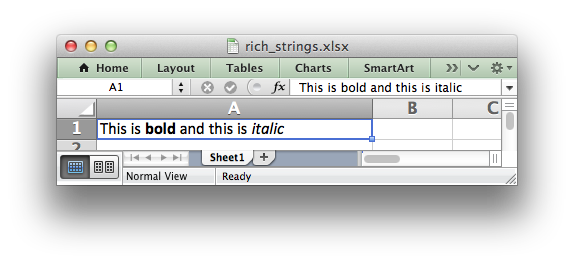Both row-column and A1 style notation are supported. The following are equivalent:

```worksheet.write_rich_string(0, 0, 'This is ', bold, 'bold')
worksheet.write_rich_string('A1', 'This is ', bold, 'bold')
```

See Working with Cell Notation for more details.

The basic rule is to break the string into fragments and put a `Format` object before the fragment that you want to format. For example:

```# Unformatted string.
'This is an example string'

# Break it into fragments.
'This is an ', 'example', ' string'

# Add formatting before the fragments you want formatted.
'This is an ', format, 'example', ' string'

# In XlsxWriter.
worksheet.write_rich_string('A1',
'This is an ', format, 'example', ' string')
```

String fragments that don’t have a format are given a default format. So for example when writing the string “Some bold text” you would use the first example below but it would be equivalent to the second:

```# Some bold format and a default format.

# With default formatting:
worksheet.write_rich_string('A1',
'Some ',
bold, 'bold',
' text')

# Or more explicitly:
worksheet.write_rich_string('A1',
default, 'Some ',
bold,    'bold',
default, ' text')
```

If you have formats and segments in a list you can add them like this, using the standard Python list unpacking syntax:

```segments = ['This is ', bold, 'bold', ' and this is ', blue, 'blue']
worksheet.write_rich_string('A9', *segments)
```

In Excel only the font properties of the format such as font name, style, size, underline, color and effects are applied to the string fragments in a rich string. Other features such as border, background, text wrap and alignment must be applied to the cell.

The `write_rich_string()` method allows you to do this by using the last argument as a cell format (if it is a format object). The following example centers a rich string in the cell:

```bold   = workbook.add_format({'bold': True})

worksheet.write_rich_string('A5',
'Some ',
bold, 'bold text',
' centered',
center)
```

Note

Excel doesn’t allow the use of two consecutive formats in a rich string or an empty string fragment. For either of these conditions a warning is raised and the input to `write_rich_string()` is ignored.

Also, the maximum string size supported by Excel is 32,767 characters. If the rich string exceeds this limit a warning is raised and the input to `write_rich_string()` is ignored.

## worksheet.write_row()

`write_row`(row, col, data[, cell_format])

Write a row of data starting from (row, col).

Parameters: row (int) – The cell row (zero indexed). col (int) – The cell column (zero indexed). data – Cell data to write. Variable types. cell_format (Format) – Optional Format object. 0: Success. Other: Error return value of the `write()` method.

The `write_row()` method can be used to write a list of data in one go. This is useful for converting the results of a database query into an Excel worksheet. The `write()` method is called for each element of the data. For example:

```# Some sample data.
data = ('Foo', 'Bar', 'Baz')

# Write the data to a sequence of cells.
worksheet.write_row('A1', data)

# The above example is equivalent to:
worksheet.write('A1', data)
worksheet.write('B1', data)
worksheet.write('C1', data)
```

Both row-column and A1 style notation are supported. The following are equivalent:

```worksheet.write_row(0, 0, data)
worksheet.write_row('A1', data)
```

See Working with Cell Notation for more details.

## worksheet.write_column()

`write_column`(row, col, data[, cell_format])

Write a column of data starting from (row, col).

Parameters: row (int) – The cell row (zero indexed). col (int) – The cell column (zero indexed). data – Cell data to write. Variable types. cell_format (Format) – Optional Format object. 0: Success. Other: Error return value of the `write()` method.

The `write_column()` method can be used to write a list of data in one go. This is useful for converting the results of a database query into an Excel worksheet. The `write()` method is called for each element of the data. For example:

```# Some sample data.
data = ('Foo', 'Bar', 'Baz')

# Write the data to a sequence of cells.
worksheet.write_column('A1', data)

# The above example is equivalent to:
worksheet.write('A1', data)
worksheet.write('A2', data)
worksheet.write('A3', data)
```

Both row-column and A1 style notation are supported. The following are equivalent:

```worksheet.write_column(0, 0, data)
worksheet.write_column('A1', data)
```

See Working with Cell Notation for more details.

## worksheet.set_row()

`set_row`(row, height, cell_format, options)

Set properties for a row of cells.

Parameters: row (int) – The worksheet row (zero indexed). height (float) – The row height, in character units. cell_format (Format) – Optional Format object. options (dict) – Optional row parameters: hidden, level, collapsed. 0: Success. -1: Row is out of worksheet bounds.

The `set_row()` method is used to change the default properties of a row. The most common use for this method is to change the height of a row:

```worksheet.set_row(0, 20)  # Set the height of Row 1 to 20.
```

The height is specified in character units. To specify the height in pixels use the `set_row_pixels()` method.

The other common use for `set_row()` is to set the Format for all cells in the row:

```cell_format = workbook.add_format({'bold': True})

worksheet.set_row(0, 20, cell_format)
```

If you wish to set the format of a row without changing the default row height you can pass `None` as the height parameter or use the default row height of 15:

```worksheet.set_row(1, None, cell_format)
worksheet.set_row(1, 15,   cell_format)  # Same as above.
```

The `cell_format` parameter will be applied to any cells in the row that don’t have a format. As with Excel it is overridden by an explicit cell format. For example:

```worksheet.set_row(0, None, format1)      # Row 1 has format1.

worksheet.write('A1', 'Hello')           # Cell A1 defaults to format1.
worksheet.write('B1', 'Hello', format2)  # Cell B1 keeps format2.
```

The `options` parameter is a dictionary with the following possible keys:

• `'hidden'`
• `'level'`
• `'collapsed'`

Options can be set as follows:

```worksheet.set_row(0, 20, cell_format, {'hidden': True})

# Or use defaults for other properties and set the options only.
worksheet.set_row(0, None, None, {'hidden': True})
```

The `'hidden'` option is used to hide a row. This can be used, for example, to hide intermediary steps in a complicated calculation:

```worksheet.set_row(0, 20, cell_format, {'hidden': True})
```

The `'level'` parameter is used to set the outline level of the row. Outlines are described in Working with Outlines and Grouping. Adjacent rows with the same outline level are grouped together into a single outline.

The following example sets an outline level of 1 for some rows:

```worksheet.set_row(0, None, None, {'level': 1})
worksheet.set_row(1, None, None, {'level': 1})
worksheet.set_row(2, None, None, {'level': 1})
```

Excel allows up to 7 outline levels. The `'level'` parameter should be in the range `0 <= level <= 7`.

The `'hidden'` parameter can also be used to hide collapsed outlined rows when used in conjunction with the `'level'` parameter:

```worksheet.set_row(1, None, None, {'hidden': 1, 'level': 1})
worksheet.set_row(2, None, None, {'hidden': 1, 'level': 1})
```

The `'collapsed'` parameter is used in collapsed outlines to indicate which row has the collapsed `'+'` symbol:

```worksheet.set_row(3, None, None, {'collapsed': 1})
```

## worksheet.set_row_pixels()

`set_row_pixels`(row, height, cell_format, options)

Set properties for a row of cells, with the row height in pixels.

Parameters: row (int) – The worksheet row (zero indexed). height (float) – The row height, in pixels. cell_format (Format) – Optional Format object. options (dict) – Optional row parameters: hidden, level, collapsed. 0: Success. -1: Row is out of worksheet bounds.

The `set_row_pixels()` method is identical to `set_row()` except that the height can be set in pixels instead of Excel character units:

```worksheet.set_row_pixels(0, 18)  # Same as 24 in character units.
```

All other parameters and options are the same as `set_row()`. See the documentation on `set_row()` for more details.

## worksheet.set_column()

`set_column`(first_col, last_col, width, cell_format, options)

Set properties for one or more columns of cells.

Parameters: first_col (int) – First column (zero-indexed). last_col (int) – Last column (zero-indexed). Can be same as first_col. width (float) – The width of the column(s), in character units. cell_format (Format) – Optional Format object. options (dict) – Optional parameters: hidden, level, collapsed. 0: Success. -1: Column is out of worksheet bounds.

The `set_column()` method can be used to change the default properties of a single column or a range of columns:

```worksheet.set_column(1, 3, 30)  # Width of columns B:D set to 30.
```

If `set_column()` is applied to a single column the value of `first_col` and `last_col` should be the same:

```worksheet.set_column(1, 1, 30)  # Width of column B set to 30.
```

It is also possible, and generally clearer, to specify a column range using the form of A1 notation used for columns. See Working with Cell Notation for more details.

Examples:

```worksheet.set_column(0, 0, 20)   # Column  A   width set to 20.
worksheet.set_column(1, 3, 30)   # Columns B-D width set to 30.
worksheet.set_column('E:E', 20)  # Column  E   width set to 20.
worksheet.set_column('F:H', 30)  # Columns F-H width set to 30.
```

The `width` parameter sets the column width in the same units used by Excel which is: the number of characters in the default font. The default width is 8.43 in the default font of Calibri 11. The actual relationship between a string width and a column width in Excel is complex. See the following explanation of column widths from the Microsoft support documentation for more details. To set the width in pixels use the `set_column_pixels()` method.

See also the `autofit()` method for simulated autofitting of column widths.

As usual the `cell_format` Format parameter is optional. If you wish to set the format without changing the default column width you can pass `None` as the width parameter:

```cell_format = workbook.add_format({'bold': True})

worksheet.set_column(0, 0, None, cell_format)
```

The `cell_format` parameter will be applied to any cells in the column that don’t have a format. For example:

```worksheet.set_column('A:A', None, format1)  # Col 1 has format1.

worksheet.write('A1', 'Hello')              # Cell A1 defaults to format1.
worksheet.write('A2', 'Hello', format2)     # Cell A2 keeps format2.
```

A row format takes precedence over a default column format:

```worksheet.set_row(0, None, format1)         # Set format for row 1.
worksheet.set_column('A:A', None, format2)  # Set format for col 1.

worksheet.write('A1', 'Hello')              # Defaults to format1
worksheet.write('A2', 'Hello')              # Defaults to format2
```

The `options` parameter is a dictionary with the following possible keys:

• `'hidden'`
• `'level'`
• `'collapsed'`

Options can be set as follows:

```worksheet.set_column('D:D', 20, cell_format, {'hidden': 1})

# Or use defaults for other properties and set the options only.
worksheet.set_column('E:E', None, None, {'hidden': 1})
```

The `'hidden'` option is used to hide a column. This can be used, for example, to hide intermediary steps in a complicated calculation:

```worksheet.set_column('D:D', 20,  cell_format, {'hidden': 1})
```

The `'level'` parameter is used to set the outline level of the column. Outlines are described in Working with Outlines and Grouping. Adjacent columns with the same outline level are grouped together into a single outline.

The following example sets an outline level of 1 for columns B to G:

```worksheet.set_column('B:G', None, None, {'level': 1})
```

Excel allows up to 7 outline levels. The `'level'` parameter should be in the range `0 <= level <= 7`.

The `'hidden'` parameter can also be used to hide collapsed outlined columns when used in conjunction with the `'level'` parameter:

```worksheet.set_column('B:G', None, None, {'hidden': 1, 'level': 1})
```

The `'collapsed'` parameter is used in collapsed outlines to indicate which column has the collapsed `'+'` symbol:

```worksheet.set_column('H:H', None, None, {'collapsed': 1})
```

## worksheet.set_column_pixels()

`set_column_pixels`(first_col, last_col, width, cell_format, options)

Set properties for one or more columns of cells, with the width in pixels.

Parameters: first_col (int) – First column (zero-indexed). last_col (int) – Last column (zero-indexed). Can be same as first_col. width (float) – The width of the column(s), in pixels. cell_format (Format) – Optional Format object. options (dict) – Optional parameters: hidden, level, collapsed. 0: Success. -1: Column is out of worksheet bounds.

The `set_column_pixels()` method is identical to `set_column()` except that the width can be set in pixels instead of Excel character units:

```worksheet.set_column_pixels(5, 5, 75)  # Same as 10 character units.
```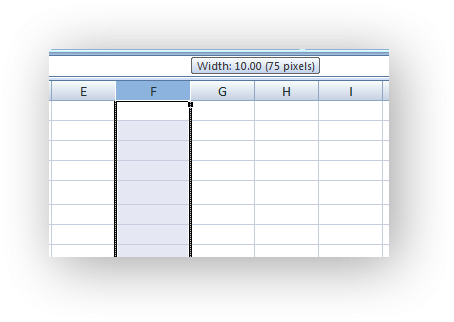All other parameters and options are the same as `set_column()`. See the documentation on `set_column()` for more details.

## worksheet.autofit()

`autofit`()

Simulates autofit for column widths.

Returns: Nothing.

The `autofit()` method can be used to simulate autofitting column widths based on the largest string/number in the column:

```worksheet.autofit()
```There is no option in the xlsx file format that can be used to say “autofit columns on loading”. Auto-fitting of columns is something that Excel does at runtime when it has access to all of the worksheet information as well as the Windows functions for calculating display areas based on fonts and formatting.

The `worksheet.autofit()` method simulates this behavior by calculating string widths using metrics taken from Excel. As such there are some limitations to be aware of when using this method:

• It is a simulated method and may not be accurate in all cases.
• It is based on the default font and font size of Calibri 11. It will not give accurate results for other fonts or font sizes.

This isn’t perfect but for most cases it should be sufficient and if not you can set your own widths, see below.

The `autofit()` method won’t override a user defined column width set with `set_column()` or `set_column_pixels()` if it is greater than the autofit value. This allows the user to set a minimum width value for a column.

You can also call `set_column()` and `set_column_pixels()` after `autofit()` to override any of the calculated values.

## worksheet.insert_image()

`insert_image`(row, col, filename[, options])

Insert an image in a worksheet cell.

Parameters: row (int) – The cell row (zero indexed). col (int) – The cell column (zero indexed). filename – Image filename (with path if required). options (dict) – Optional parameters for image position, scale and url. 0: Success. -1: Row or column is out of worksheet bounds.

This method can be used to insert a image into a worksheet. The image can be in PNG, JPEG, GIF, BMP, WMF or EMF format (see the notes about BMP and EMF below):

```worksheet.insert_image('B2', 'python.png')
```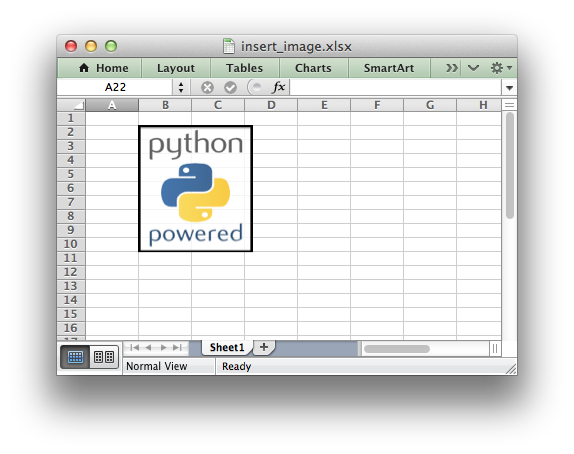Both row-column and A1 style notation are supported. The following are equivalent:

```worksheet.insert_image(1, 1, 'python.png')
worksheet.insert_image('B2', 'python.png')
```

See Working with Cell Notation for more details.

A file path can be specified with the image name:

```worksheet1.insert_image('B10', '../images/python.png')
worksheet2.insert_image('B20', r'c:\images\python.png')
```

The `insert_image()` method takes optional parameters in a dictionary to position and scale the image. The available parameters with their default values are:

```{
'x_offset':        0,
'y_offset':        0,
'x_scale':         1,
'y_scale':         1,
'object_position': 2,
'image_data':      None,
'url':             None,
'description':     None,
'decorative':      False,
}
```

The offset values are in pixels:

```worksheet1.insert_image('B2', 'python.png', {'x_offset': 15, 'y_offset': 10})
```

The offsets can be greater than the width or height of the underlying cell. This can be occasionally useful if you wish to align two or more images relative to the same cell.

The `x_scale` and `y_scale` parameters can be used to scale the image horizontally and vertically:

```worksheet.insert_image('B3', 'python.png', {'x_scale': 0.5, 'y_scale': 0.5})
```

The `url` parameter can used to add a hyperlink/url to the image. The `tip` parameter gives an optional mouseover tooltip for images with hyperlinks:

```worksheet.insert_image('B4', 'python.png', {'url': 'https://python.org'})
```

See also `write_url()` for details on supported URIs.

The `image_data` parameter is used to add an in-memory byte stream in `io.BytesIO` format:

```worksheet.insert_image('B5', 'python.png', {'image_data': image_data})
```

This is generally used for inserting images from URLs:

```url = 'https://python.org/logo.png'

worksheet.insert_image('B5', url, {'image_data': image_data})
```

When using the `image_data` parameter a filename must still be passed to `insert_image()` since it is used by Excel as a default description field (see below). However, it can be a blank string if the description isn’t required. In the previous example the filename/description is extracted from the URL string. See also Example: Inserting images from a URL or byte stream into a worksheet.

The `description` field can be used to specify a description or “alt text” string for the image. In general this would be used to provide a text description of the image to help accessibility. It is an optional parameter and defaults to the filename of the image. It can be used as follows:

```worksheet.insert_image('B3', 'python.png',
{'description': 'The logo of the Python programming language.'})
```The optional `decorative` parameter is also used to help accessibility. It is used to mark the image as decorative, and thus uninformative, for automated screen readers. As in Excel, if this parameter is in use the `description` field isn’t written. It is used as follows:

```worksheet.insert_image('B3', 'python.png', {'decorative': True})
```

The `object_position` parameter can be used to control the object positioning of the image:

```worksheet.insert_image('B3', 'python.png', {'object_position': 1})
```

Where `object_position` has the following allowable values:

1. Move and size with cells.
2. Move but don’t size with cells (the default).
3. Don’t move or size with cells.
4. Same as Option 1 to “move and size with cells” except XlsxWriter applies hidden cells after the image is inserted.

See Working with Object Positioning for more detailed information about the positioning and scaling of images within a worksheet.

Note

• BMP images are only supported for backward compatibility. In general it is best to avoid BMP images since they aren’t compressed. If used, BMP images must be 24 bit, true color, bitmaps.
• EMF images can have very small differences in width and height when compared to Excel files. Despite a lot of effort and testing it wasn’t possible to exactly match Excel’s calculations for handling the dimensions of EMF files. However, the differences are small (< 1%) and in general aren’t visible.

## worksheet.insert_chart()

`insert_chart`(row, col, chart[, options])

Write a string to a worksheet cell.

Parameters: row (int) – The cell row (zero indexed). col (int) – The cell column (zero indexed). chart – A chart object. options (dict) – Optional parameters to position and scale the chart. 0: Success. -1: Row or column is out of worksheet bounds.

This method can be used to insert a chart into a worksheet. A chart object is created via the Workbook `add_chart()` method where the chart type is specified:

```chart = workbook.add_chart({type, 'column'})
```

It is then inserted into a worksheet as an embedded chart:

```worksheet.insert_chart('B5', chart)
```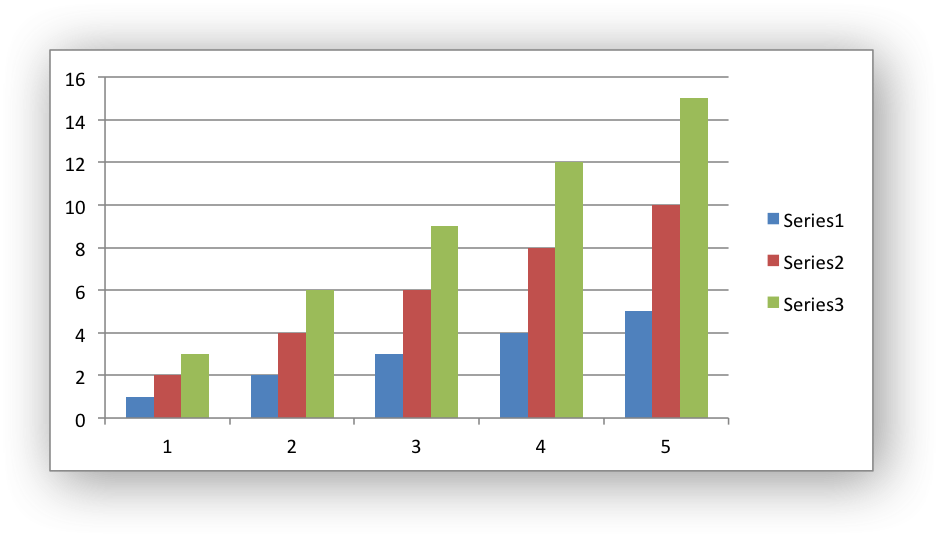Note

A chart can only be inserted into a worksheet once. If several similar charts are required then each one must be created separately with `add_chart()`.

Both row-column and A1 style notation are supported. The following are equivalent:

```worksheet.insert_chart(4, 1, chart)
worksheet.insert_chart('B5', chart)
```

See Working with Cell Notation for more details.

The `insert_chart()` method takes optional parameters in a dictionary to position and scale the chart. The available parameters with their default values are:

```{
'x_offset':        0,
'y_offset':        0,
'x_scale':         1,
'y_scale':         1,
'object_position': 1,
'description':     None,
'decorative':      False,
}
```

The offset values are in pixels:

```worksheet.insert_chart('B5', chart, {'x_offset': 25, 'y_offset': 10})
```

The `x_scale` and `y_scale` parameters can be used to scale the chart horizontally and vertically:

```worksheet.insert_chart('B5', chart, {'x_scale': 0.5, 'y_scale': 0.5})
```

These properties can also be set via the Chart `set_size()` method.

The `description` field can be used to specify a description or “alt text” string for the chart. In general this would be used to provide a text description of the chart to help accessibility. It is an optional parameter and has no default. It can be used as follows:

```worksheet.insert_chart('B5', chart,
{'description': 'Chart showing sales for the current year'})
```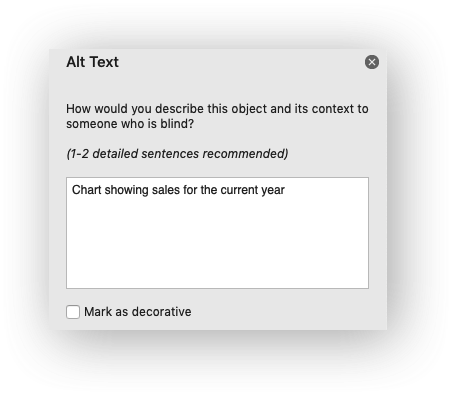The optional `decorative` parameter is also used to help accessibility. It is used to mark the chart as decorative, and thus uninformative, for automated screen readers. As in Excel, if this parameter is in use the `description` field isn’t written. It is used as follows:

```worksheet.insert_chart('B5', chart, {'decorative': True})
```

The `object_position` parameter can be used to control the object positioning of the chart:

```worksheet.insert_chart('B5', chart, {'object_position': 2})
```

Where `object_position` has the following allowable values:

1. Move and size with cells (the default).
2. Move but don’t size with cells.
3. Don’t move or size with cells.

See Working with Object Positioning for more detailed information about the positioning and scaling of charts within a worksheet.

## worksheet.insert_textbox()

`insert_textbox`(row, col, textbox[, options])

Write a string to a worksheet cell.

Parameters: row (int) – The cell row (zero indexed). col (int) – The cell column (zero indexed). text (string) – The text in the textbox. options (dict) – Optional parameters to position and scale the textbox. 0: Success. -1: Row or column is out of worksheet bounds.

This method can be used to insert a textbox into a worksheet:

```worksheet.insert_textbox('B2', 'A simple textbox with some text')
```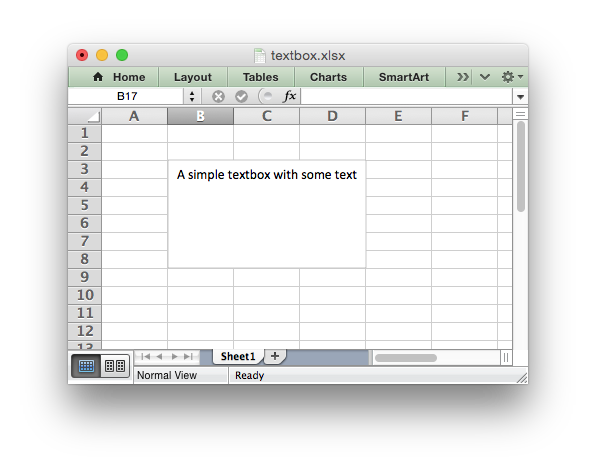Both row-column and A1 style notation are supported. The following are equivalent:

```worksheet.insert_textbox(1, 1, 'Some text')
worksheet.insert_textbox('B2', 'Some text')
```

See Working with Cell Notation for more details.

The size and formatting of the textbox can be controlled via the `options` dict:

```# Size and position
width
height
x_scale
y_scale
x_offset
y_offset
object_position

# Formatting
line
border
fill
font
align
text_rotation

url
tip

# Accessibility
description
decorative
```

These options are explained in more detail in the Working with Textboxes section.

See Working with Object Positioning for more detailed information about the positioning and scaling of images within a worksheet.

## worksheet.insert_button()

`insert_button`(row, col[, options])

Insert a VBA button control on a worksheet.

Parameters: row (int) – The cell row (zero indexed). col (int) – The cell column (zero indexed). options (dict) – Optional parameters to position and scale the button. 0: Success. -1: Row or column is out of worksheet bounds.

The `insert_button()` method can be used to insert an Excel form button into a worksheet.

This method is generally only useful when used in conjunction with the Workbook `add_vba_project()` method to tie the button to a macro from an embedded VBA project:

```# Add the VBA project binary.

# Add a button tied to a macro in the VBA project.
worksheet.insert_button('B3', {'macro':   'say_hello',
'caption': 'Press Me'})
```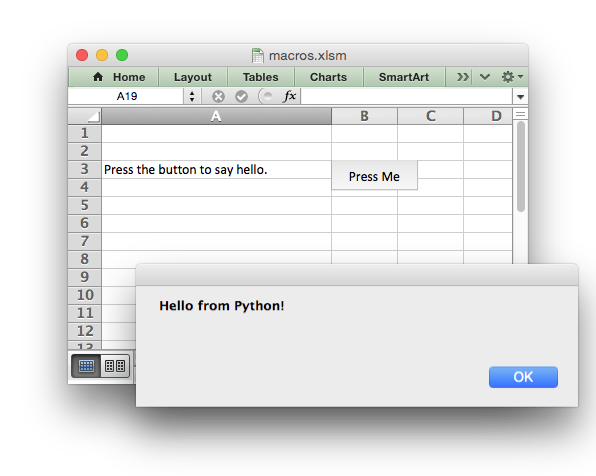See Working with VBA Macros and Example: Adding a VBA macro to a Workbook for more details.

Both row-column and A1 style notation are supported. The following are equivalent:

```worksheet.insert_button(2, 1, {'macro':   'say_hello',
'caption': 'Press Me'})

worksheet.insert_button('B3', {'macro':   'say_hello',
'caption': 'Press Me'})
```

See Working with Cell Notation for more details.

The `insert_button()` method takes optional parameters in a dictionary to position and scale the chart. The available parameters with their default values are:

```{
'macro':       None,
'caption':     'Button 1',
'width':       64,
'height':      20.
'x_offset':    0,
'y_offset':    0,
'x_scale':     1,
'y_scale':     1,
'description': None,
}
```

The `macro` option is used to set the macro that the button will invoke when the user clicks on it. The macro should be included using the Workbook `add_vba_project()` method shown above.

The `caption` is used to set the caption on the button. The default is `Button n` where `n` is the button number.

The default button `width` is 64 pixels which is the width of a default cell and the default button `height` is 20 pixels which is the height of a default cell.

The offset, scale and description options are the same as for `insert_chart()`, see above.

## worksheet.data_validation()

`data_validation`(first_row, first_col, last_row, last_col, options)

Write a conditional format to range of cells.

Parameters: first_row (int) – The first row of the range. (All zero indexed.) first_col (int) – The first column of the range. last_row (int) – The last row of the range. last_col (int) – The last col of the range. options (dict) – Data validation options. 0: Success. -1: Row or column is out of worksheet bounds. -2: Incorrect parameter or option.

The `data_validation()` method is used to construct an Excel data validation or to limit the user input to a dropdown list of values:

```worksheet.data_validation('B3', {'validate': 'integer',
'criteria': 'between',
'minimum': 1,
'maximum': 10})

worksheet.data_validation('B13', {'validate': 'list',
'source': ['open', 'high', 'close']})
```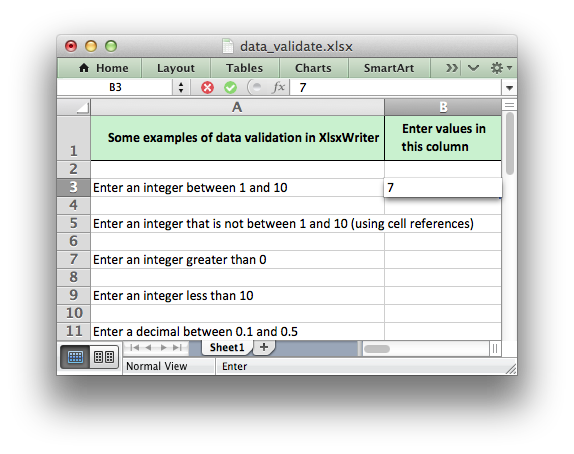The data validation can be applied to a single cell or a range of cells. As usual you can use A1 or Row/Column notation, see Working with Cell Notation:

```worksheet.data_validation(1, 1, {'validate': 'list',
'source': ['open', 'high', 'close']})

worksheet.data_validation('B2', {'validate': 'list',
'source': ['open', 'high', 'close']})
```

With Row/Column notation you must specify all four cells in the range: `(first_row, first_col, last_row, last_col)`. If you need to refer to a single cell set the last_ values equal to the first_ values. With A1 notation you can refer to a single cell or a range of cells:

```worksheet.data_validation(0, 0, 4, 1, {...})
worksheet.data_validation('B1',       {...})
worksheet.data_validation('C1:E5',    {...})
```

The options parameter in `data_validation()` must be a dictionary containing the parameters that describe the type and style of the data validation. There are a lot of available options which are described in detail in a separate section: Working with Data Validation. See also Example: Data Validation and Drop Down Lists.

## worksheet.conditional_format()

`conditional_format`(first_row, first_col, last_row, last_col, options)

Write a conditional format to range of cells.

Parameters: first_row (int) – The first row of the range. (All zero indexed.) first_col (int) – The first column of the range. last_row (int) – The last row of the range. last_col (int) – The last col of the range. options (dict) – Conditional formatting options. 0: Success. -1: Row or column is out of worksheet bounds. -2: Incorrect parameter or option.

The `conditional_format()` method is used to add formatting to a cell or range of cells based on user defined criteria:

```worksheet.conditional_format('B3:K12', {'type':     'cell',
'criteria': '>=',
'value':    50,
'format':   format1})
```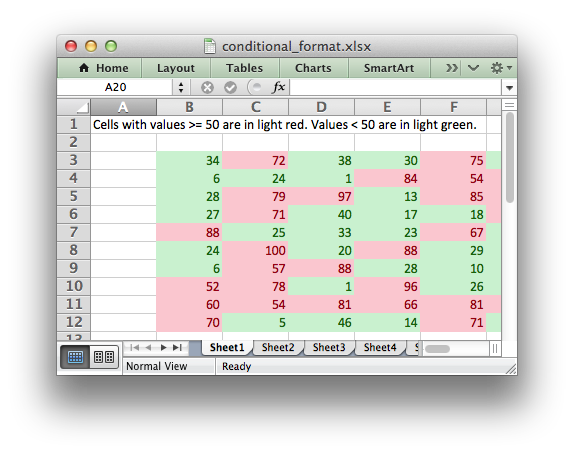The conditional format can be applied to a single cell or a range of cells. As usual you can use A1 or Row/Column notation, see Working with Cell Notation:

```worksheet.conditional_format(0, 0, 2, 1, {'type':     'cell',
'criteria': '>=',
'value':    50,
'format':   format1})

# This is equivalent to the following:
worksheet.conditional_format('A1:B3', {'type':     'cell',
'criteria': '>=',
'value':    50,
'format':   format1})
```

With Row/Column notation you must specify all four cells in the range: `(first_row, first_col, last_row, last_col)`. If you need to refer to a single cell set the last_ values equal to the first_ values. With A1 notation you can refer to a single cell or a range of cells:

```worksheet.conditional_format(0, 0, 4, 1, {...})
worksheet.conditional_format('B1',       {...})
worksheet.conditional_format('C1:E5',    {...})
```

The options parameter in `conditional_format()` must be a dictionary containing the parameters that describe the type and style of the conditional format. There are a lot of available options which are described in detail in a separate section: Working with Conditional Formatting. See also Example: Conditional Formatting.

`add_table`(first_row, first_col, last_row, last_col, options)

Add an Excel table to a worksheet.

Parameters: first_row (int) – The first row of the range. (All zero indexed.) first_col (int) – The first column of the range. last_row (int) – The last row of the range. last_col (int) – The last col of the range. options (dict) – Table formatting options. (Optional) OverlappingRange – if the range overlaps a previous merge or table range. 0: Success. -1: Row or column is out of worksheet bounds. -2: Incorrect parameter or option. -3: Not supported in `constant_memory` mode.

The `add_table()` method is used to group a range of cells into an Excel Table:

```worksheet.add_table('B3:F7', { ... })
```

This method contains a lot of parameters and is described in Working with Worksheet Tables.

Both row-column and A1 style notation are supported. The following are equivalent:

```worksheet.add_table(2, 1, 6, 5, { ... })
```

See Working with Cell Notation for more details.

Note

Tables aren’t available in XlsxWriter when `Workbook()` `'constant_memory'` mode is enabled.

`add_sparkline`(row, col, options)
Parameters: row (int) – The cell row (zero indexed). col (int) – The cell column (zero indexed). options (dict) – Sparkline formatting options. 0: Success. -1: Row or column is out of worksheet bounds. -2: Incorrect parameter or option.

Sparklines are small charts that fit in a single cell and are used to show trends in data.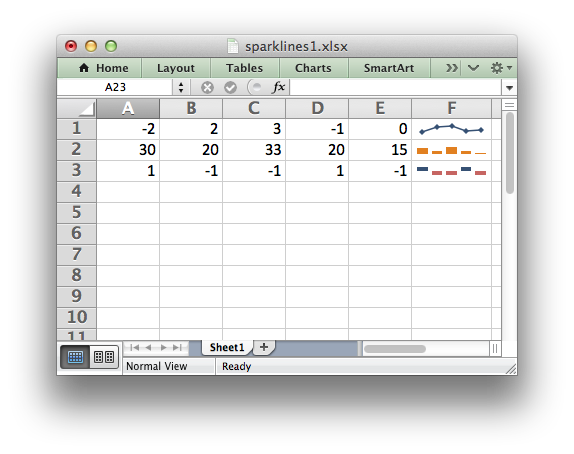The `add_sparkline()` worksheet method is used to add sparklines to a cell or a range of cells:

```worksheet.add_sparkline('F1', {'range': 'A1:E1'})
```

Both row-column and A1 style notation are supported. The following are equivalent:

```worksheet.add_sparkline(0, 5, {'range': 'A1:E1'})
```

See Working with Cell Notation for more details.

This method contains a lot of parameters and is described in detail in Working with Sparklines.

Note

Sparklines are a feature of Excel 2010+ only. You can write them to an XLSX file that can be read by Excel 2007 but they won’t be displayed.

## worksheet.write_comment()

`write_comment`(row, col, comment[, options])

Write a comment to a worksheet cell.

Parameters: row (int) – The cell row (zero indexed). col (int) – The cell column (zero indexed). comment (string) – String to write to cell. options (dict) – Comment formatting options. 0: Success. -1: Row or column is out of worksheet bounds. -2: String longer than 32k characters.

The `write_comment()` method is used to add a comment to a cell. A comment is indicated in Excel by a small red triangle in the upper right-hand corner of the cell. Moving the cursor over the red triangle will reveal the comment.

The following example shows how to add a comment to a cell:

```worksheet.write('A1', 'Hello')
worksheet.write_comment('A1', 'This is a comment')
```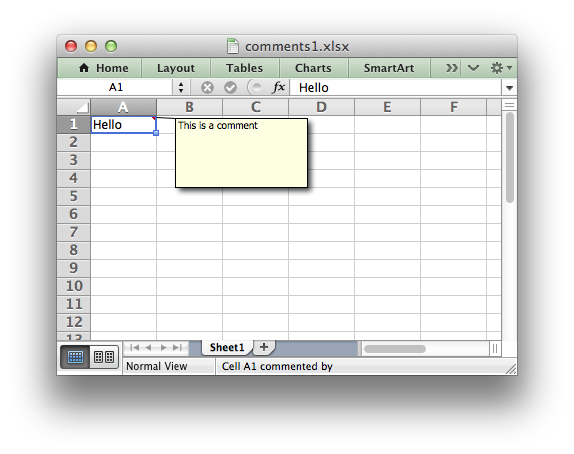Both row-column and A1 style notation are supported. The following are equivalent:

```worksheet.write_comment(0, 0, 'This is a comment')
worksheet.write_comment('A1', 'This is a comment')
```

See Working with Cell Notation for more details.

The properties of the cell comment can be modified by passing an optional dictionary of key/value pairs to control the format of the comment. For example:

```worksheet.write_comment('C3', 'Hello', {'x_scale': 1.2, 'y_scale': 0.8})
```

Most of these options are quite specific and in general the default comment behavior will be all that you need. However, should you need greater control over the format of the cell comment the following options are available:

```author
visible
x_scale
width
y_scale
height
color
font_name
font_size
start_cell
start_row
start_col
x_offset
y_offset
```

`show_comments`()

Make any comments in the worksheet visible.

This method is used to make all cell comments visible when a worksheet is opened:

```worksheet.show_comments()
```

Individual comments can be made visible using the `visible` parameter of the `write_comment` method (see above):

```worksheet.write_comment('C3', 'Hello', {'visible': True})
```

If all of the cell comments have been made visible you can hide individual comments as follows:

```worksheet.show_comments()
worksheet.write_comment('C3', 'Hello', {'visible': False})
```

`set_comments_author`(author)

Set the default author of the cell comments.

Parameters: author (string) – Comment author.

This method is used to set the default author of all cell comments:

```worksheet.set_comments_author('John Smith')
```

Individual comment authors can be set using the `author` parameter of the `write_comment` method (see above).

If no author is specified the default comment author name is an empty string.

## worksheet.get_name()

`get_name`()

Retrieve the worksheet name.

The `get_name()` method is used to retrieve the name of a worksheet. This is something useful for debugging or logging:

```for worksheet in workbook.worksheets():
print worksheet.get_name()
```

There is no `set_name()` method. The only safe way to set the worksheet name is via the `add_worksheet()` method.

## worksheet.activate()

`activate`()

Make a worksheet the active, i.e., visible worksheet.

The `activate()` method is used to specify which worksheet is initially visible in a multi-sheet workbook:

```worksheet1 = workbook.add_worksheet()

worksheet3.activate()
```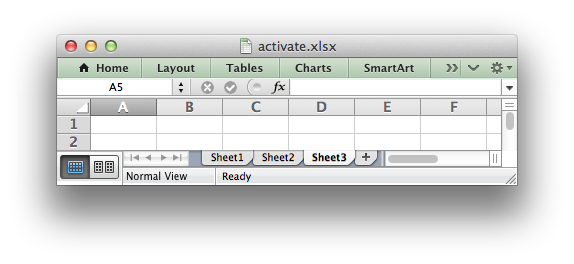More than one worksheet can be selected via the `select()` method, see below, however only one worksheet can be active.

The default active worksheet is the first worksheet.

## worksheet.select()

`select`()

Set a worksheet tab as selected.

The `select()` method is used to indicate that a worksheet is selected in a multi-sheet workbook:

```worksheet1.activate()
worksheet2.select()
worksheet3.select()
```

A selected worksheet has its tab highlighted. Selecting worksheets is a way of grouping them together so that, for example, several worksheets could be printed in one go. A worksheet that has been activated via the `activate()` method will also appear as selected.

## worksheet.hide()

`hide`()

Hide the current worksheet.

The `hide()` method is used to hide a worksheet:

```worksheet2.hide()
```

You may wish to hide a worksheet in order to avoid confusing a user with intermediate data or calculations.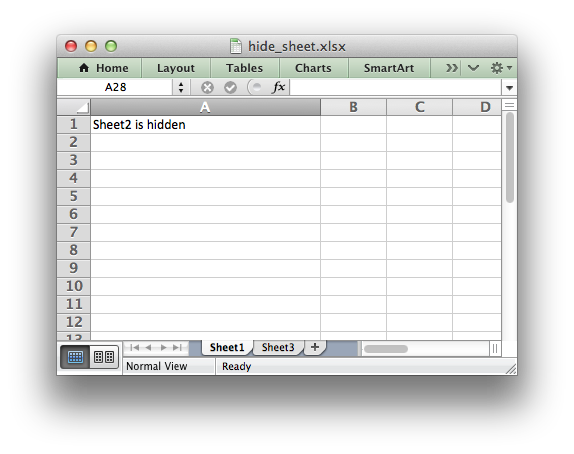A hidden worksheet can not be activated or selected so this method is mutually exclusive with the `activate()` and `select()` methods. In addition, since the first worksheet will default to being the active worksheet, you cannot hide the first worksheet without activating another sheet:

```worksheet2.activate()
worksheet1.hide()
```

See Example: Hiding Worksheets for more details.

## worksheet.set_first_sheet()

`set_first_sheet`()

Set current worksheet as the first visible sheet tab.

The `activate()` method determines which worksheet is initially selected. However, if there are a large number of worksheets the selected worksheet may not appear on the screen. To avoid this you can select which is the leftmost visible worksheet tab using `set_first_sheet()`:

```for in range(1, 21):

worksheet19.set_first_sheet()  # First visible worksheet tab.
worksheet20.activate()         # First visible worksheet.
```

This method is not required very often. The default value is the first worksheet.

## worksheet.merge_range()

`merge_range`(first_row, first_col, last_row, last_col, data[, cell_format])

Merge a range of cells.

Parameters: first_row (int) – The first row of the range. (All zero indexed.) first_col (int) – The first column of the range. last_row (int) – The last row of the range. last_col (int) – The last col of the range. data – Cell data to write. Variable types. cell_format (Format) – Optional Format object. OverlappingRange – if the range overlaps a previous merge or table range. 0: Success. -1: Row or column is out of worksheet bounds. Other: Error return value of the called `write()` method.

The `merge_range()` method allows cells to be merged together so that they act as a single area.

Excel generally merges and centers cells at same time. To get similar behavior with XlsxWriter you need to apply a Format:

```merge_format = workbook.add_format({'align': 'center'})

worksheet.merge_range('B3:D4', 'Merged Cells', merge_format)
```

Both row-column and A1 style notation are supported. The following are equivalent:

```worksheet.merge_range(2, 1, 3, 3, 'Merged Cells', merge_format)
worksheet.merge_range('B3:D4',    'Merged Cells', merge_format)
```

See Working with Cell Notation for more details.

It is possible to apply other formatting to the merged cells as well:

```merge_format = workbook.add_format({
'bold':     True,
'border':   6,
'align':    'center',
'valign':   'vcenter',
'fg_color': '#D7E4BC',
})

worksheet.merge_range('B3:D4', 'Merged Cells', merge_format)
```See Example: Merging Cells for more details.

The `merge_range()` method writes its `data` argument using `write()`. Therefore it will handle numbers, strings and formulas as usual. If this doesn’t handle your data correctly then you can overwrite the first cell with a call to one of the other `write_*()` methods using the same Format as in the merged cells. See Example: Merging Cells with a Rich String.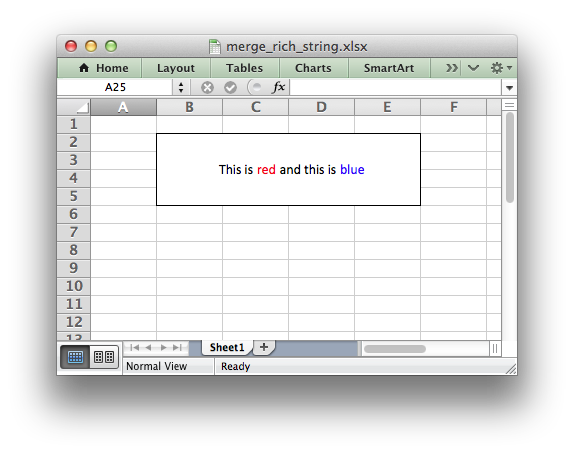Note

Merged ranges generally don’t work in XlsxWriter when `Workbook()` `'constant_memory'` mode is enabled.

## worksheet.autofilter()

`autofilter`(first_row, first_col, last_row, last_col)

Set the autofilter area in the worksheet.

Parameters: first_row (int) – The first row of the range. (All zero indexed.) first_col (int) – The first column of the range. last_row (int) – The last row of the range. last_col (int) – The last col of the range.

The `autofilter()` method allows an autofilter to be added to a worksheet. An autofilter is a way of adding drop down lists to the headers of a 2D range of worksheet data. This allows users to filter the data based on simple criteria so that some data is shown and some is hidden.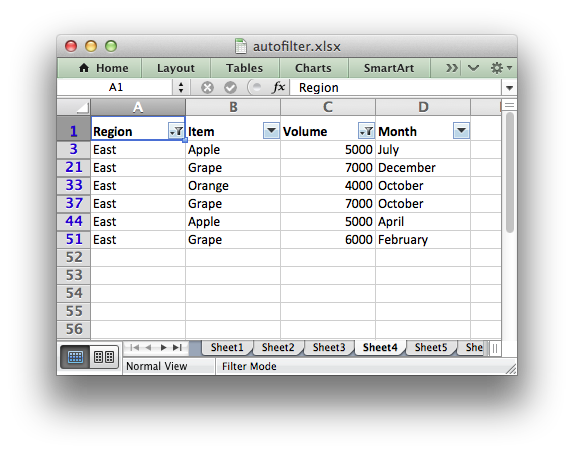To add an autofilter to a worksheet:

```worksheet.autofilter('A1:D11')
```

Both row-column and A1 style notation are supported. The following are equivalent:

```worksheet.autofilter(0, 0, 10, 3)
worksheet.autofilter('A1:D11')
```

See Working with Cell Notation for more details.

Filter conditions can be applied using the `filter_column()` or `filter_column_list()` methods.

See Working with Autofilters for more details.

## worksheet.filter_column()

`filter_column`(col, criteria)

Set the column filter criteria.

Parameters: col (int) – Filter column (zero-indexed). criteria (string) – Filter criteria.

The `filter_column` method can be used to filter columns in a autofilter range based on simple conditions.

The conditions for the filter are specified using simple expressions:

```worksheet.filter_column('A', 'x > 2000')
worksheet.filter_column('B', 'x > 2000 and x < 5000')
```

The `col` parameter can either be a zero indexed column number or a string column name:

```worksheet.filter_column(2,   'x > 2000')
worksheet.filter_column('C', 'x > 2000')
```

See Working with Cell Notation for more details.

It isn’t sufficient to just specify the filter condition. You must also hide any rows that don’t match the filter condition. See Working with Autofilters for more details.

## worksheet.filter_column_list()

`filter_column_list`(col, filters)

Set the column filter criteria in Excel 2007 list style.

Parameters: col (int) – Filter column (zero-indexed). filters (list) – List of filter criteria to match.

The `filter_column_list()` method can be used to represent filters with multiple selected criteria:

```worksheet.filter_column_list('A', ['March', 'April', 'May'])
```

The `col` parameter can either be a zero indexed column number or a string column name:

```worksheet.filter_column_list(2,   ['March', 'April', 'May'])
worksheet.filter_column_list('C', ['March', 'April', 'May'])
```

See Working with Cell Notation for more details.

One or more criteria can be selected:

```worksheet.filter_column_list('A', ['March'])
worksheet.filter_column_list('C', [100, 110, 120, 130])
```

To filter blanks as part of the list use Blanks as a list item:

```worksheet.filter_column_list('A', ['March', 'April', 'May', 'Blanks'])
```

It isn’t sufficient to just specify filters. You must also hide any rows that don’t match the filter condition. See Working with Autofilters for more details.

## worksheet.set_selection()

`set_selection`(first_row, first_col, last_row, last_col)

Set the selected cell or cells in a worksheet.

Parameters: first_row (int) – The first row of the range. (All zero indexed.) first_col (int) – The first column of the range. last_row (int) – The last row of the range. last_col (int) – The last col of the range.

The `set_selection()` method can be used to specify which cell or range of cells is selected in a worksheet. The most common requirement is to select a single cell, in which case the `first_` and `last_` parameters should be the same.

The active cell within a selected range is determined by the order in which `first_` and `last_` are specified.

Examples:

```worksheet1.set_selection(3, 3, 3, 3)  # 1. Cell D4.
worksheet2.set_selection(3, 3, 6, 6)  # 2. Cells D4 to G7.
worksheet3.set_selection(6, 6, 3, 3)  # 3. Cells G7 to D4.
worksheet4.set_selection('D4')        # Same as 1.
worksheet5.set_selection('D4:G7')     # Same as 2.
worksheet6.set_selection('G7:D4')     # Same as 3.
```

As shown above, both row-column and A1 style notation are supported. See Working with Cell Notation for more details. The default cell selection is `(0, 0)`, `'A1'`.

## worksheet.set_top_left_cell()

`set_top_left_cell`(row, col)

Set the first visible cell at the top left of a worksheet.

Parameters: row (int) – The cell row (zero indexed). col (int) – The cell column (zero indexed).

This `set_top_left_cell` method can be used to set the top leftmost visible cell in the worksheet:

```worksheet.set_top_left_cell(31, 26)

# Same as:
worksheet.set_top_left_cell('AA32')
```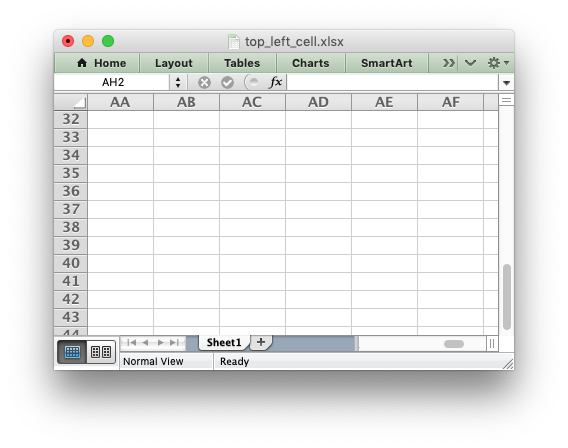As shown above, both row-column and A1 style notation are supported. See Working with Cell Notation for more details.

## worksheet.freeze_panes()

`freeze_panes`(row, col[, top_row, left_col])

Create worksheet panes and mark them as frozen.

Parameters: row (int) – The cell row (zero indexed). col (int) – The cell column (zero indexed). top_row (int) – Topmost visible row in scrolling region of pane. left_col (int) – Leftmost visible row in scrolling region of pane.

The `freeze_panes()` method can be used to divide a worksheet into horizontal or vertical regions known as panes and to “freeze” these panes so that the splitter bars are not visible.

The parameters `row` and `col` are used to specify the location of the split. It should be noted that the split is specified at the top or left of a cell and that the method uses zero based indexing. Therefore to freeze the first row of a worksheet it is necessary to specify the split at row 2 (which is 1 as the zero-based index).

You can set one of the `row` and `col` parameters as zero if you do not want either a vertical or horizontal split.

Examples:

```worksheet.freeze_panes(1, 0)  # Freeze the first row.
worksheet.freeze_panes('A2')  # Same using A1 notation.
worksheet.freeze_panes(0, 1)  # Freeze the first column.
worksheet.freeze_panes('B1')  # Same using A1 notation.
worksheet.freeze_panes(1, 2)  # Freeze first row and first 2 columns.
worksheet.freeze_panes('C2')  # Same using A1 notation.
```

As shown above, both row-column and A1 style notation are supported. See Working with Cell Notation for more details.

The parameters `top_row` and `left_col` are optional. They are used to specify the top-most or left-most visible row or column in the scrolling region of the panes. For example to freeze the first row and to have the scrolling region begin at row twenty:

```worksheet.freeze_panes(1, 0, 20, 0)
```

You cannot use A1 notation for the `top_row` and `left_col` parameters.

See Example: Freeze Panes and Split Panes for more details.

## worksheet.split_panes()

`split_panes`(x, y[, top_row, left_col])

Create worksheet panes and mark them as split.

Parameters: x (float) – The position for the vertical split. y (float) – The position for the horizontal split. top_row (int) – Topmost visible row in scrolling region of pane. left_col (int) – Leftmost visible row in scrolling region of pane.

The `split_panes` method can be used to divide a worksheet into horizontal or vertical regions known as panes. This method is different from the `freeze_panes()` method in that the splits between the panes will be visible to the user and each pane will have its own scroll bars.

The parameters `y` and `x` are used to specify the vertical and horizontal position of the split. The units for `y` and `x` are the same as those used by Excel to specify row height and column width. However, the vertical and horizontal units are different from each other. Therefore you must specify the `y` and `x` parameters in terms of the row heights and column widths that you have set or the default values which are `15` for a row and `8.43` for a column.

You can set one of the `y` and `x` parameters as zero if you do not want either a vertical or horizontal split. The parameters `top_row` and `left_col` are optional. They are used to specify the top-most or left-most visible row or column in the bottom-right pane.

Example:

```worksheet.split_panes(15, 0)     # First row.
worksheet.split_panes(0, 8.43)   # First column.
worksheet.split_panes(15, 8.43)  # First row and column.
```

You cannot use A1 notation with this method.

See Example: Freeze Panes and Split Panes for more details.

## worksheet.set_zoom()

`set_zoom`(zoom)

Set the worksheet zoom factor.

Parameters: zoom (int) – Worksheet zoom factor.

Set the worksheet zoom factor in the range `10 <= zoom <= 400`:

```worksheet1.set_zoom(50)
worksheet2.set_zoom(75)
worksheet3.set_zoom(300)
worksheet4.set_zoom(400)
```

The default zoom factor is 100. It isn’t possible to set the zoom to “Selection” because it is calculated by Excel at run-time.

Note, `set_zoom()` does not affect the scale of the printed page. For that you should use `set_print_scale()`.

## worksheet.right_to_left()

`right_to_left`()

Display the worksheet cells from right to left for some versions of Excel.

The `right_to_left()` method is used to change the default direction of the worksheet from left-to-right, with the A1 cell in the top left, to right-to-left, with the A1 cell in the top right:

```worksheet.right_to_left()
```

This is useful when creating Arabic, Hebrew or other near or far eastern worksheets that use right-to-left as the default direction.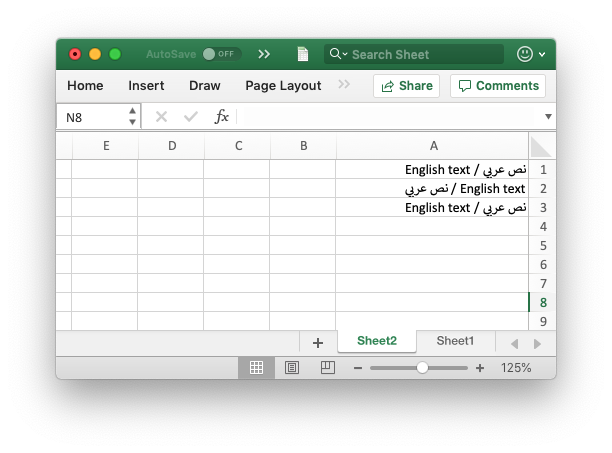See also the Format `set_reading_order()` property to set the direction of the text within cells and the Example: Left to Right worksheets and text example program.

## worksheet.hide_zero()

`hide_zero`()

Hide zero values in worksheet cells.

The `hide_zero()` method is used to hide any zero values that appear in cells:

```worksheet.hide_zero()
```

## worksheet.set_background()

`set_background`(filename[, is_byte_stream])

Set the background image for a worksheet.

Parameters: filename (str) – The image file (or byte stream). is_byte_stream (bool) – The file is a stream of bytes.

The `set_background()` method can be used to set the background image for the worksheet:

```worksheet.set_background('logo.png')
```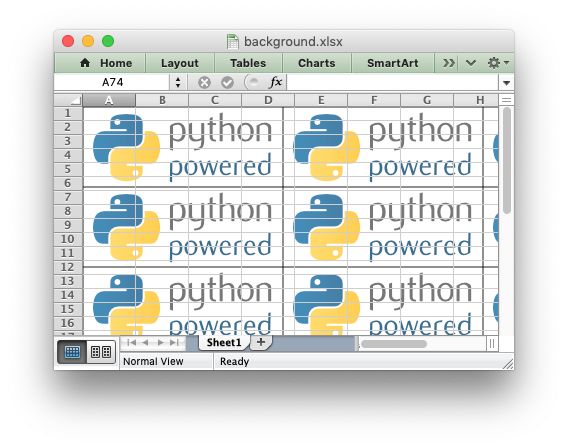The `set_background()` method supports all the image formats supported by `insert_image()`.

Some people use this method to add a watermark background to their document. However, Microsoft recommends using a header image to set a watermark. The choice of method depends on whether you want the watermark to be visible in normal viewing mode or just when the file is printed. In XlsxWriter you can get the header watermark effect using `set_header()`:

```worksheet.set_header('&C&G', {'image_center': 'watermark.png'})
```

It is also possible to pass an in-memory byte stream to `set_background()` if the `is_byte_stream` parameter is set to True. The stream should be `io.BytesIO`:

```worksheet.set_background(io_bytes, is_byte_stream=True)
```

See Example: Setting the Worksheet Background for an example.

## worksheet.set_tab_color()

`set_tab_color`()

Set the color of the worksheet tab.

Parameters: color (string) – The tab color.

The `set_tab_color()` method is used to change the color of the worksheet tab:

```worksheet1.set_tab_color('red')
worksheet2.set_tab_color('#FF9900')  # Orange
```

The color can be a Html style `#RRGGBB` string or a limited number named colors, see Working with Colors.

See Example: Setting Worksheet Tab Colors for more details.

## worksheet.protect()

`protect`()

Protect elements of a worksheet from modification.

Parameters: password (string) – A worksheet password. options (dict) – A dictionary of worksheet options to protect.

The `protect()` method is used to protect a worksheet from modification:

```worksheet.protect()
```

The `protect()` method also has the effect of enabling a cell’s `locked` and `hidden` properties if they have been set. A locked cell cannot be edited and this property is on by default for all cells. A hidden cell will display the results of a formula but not the formula itself. These properties can be set using the `set_locked()` and `set_hidden()` format methods.

```worksheet.protect('abc123')
```

The password should be an ASCII string. Passing the empty string `''` is the same as turning on protection without a password. See the note below on the “password” strength.

You can specify which worksheet elements you wish to protect by passing a dictionary in the `options` argument with any or all of the following keys:

```# Default values shown.
options = {
'objects':               False,
'scenarios':             False,
'format_cells':          False,
'format_columns':        False,
'format_rows':           False,
'insert_columns':        False,
'insert_rows':           False,
'delete_columns':        False,
'delete_rows':           False,
'select_locked_cells':   True,
'sort':                  False,
'autofilter':            False,
'pivot_tables':          False,
'select_unlocked_cells': True,
}
```

The default boolean values are shown above. Individual elements can be protected as follows:

```worksheet.protect('abc123', {'insert_rows': True})
```

For chartsheets the allowable options and default values are:

```options = {
'objects':               True,
'content':               True,
}
```

See also the `set_locked()` and `set_hidden()` format methods and Example: Enabling Cell protection in Worksheets.

Note

Worksheet level passwords in Excel offer very weak protection. They do not encrypt your data and are very easy to deactivate. Full workbook encryption is not supported by XlsxWriter. However, it is possible to encrypt an XlsxWriter file using a third party open source tool called msoffice-crypt. This works for macOS, Linux and Windows:

```msoffice-crypt.exe -e -p password clear.xlsx encrypted.xlsx
```

## worksheet.unprotect_range()

`unprotect_range`(cell_range, range_name)

Unprotect ranges within a protected worksheet.

Parameters: cell_range (string) – The cell or cell range to unprotect. range_name (string) – An name for the range.

The `unprotect_range()` method is used to unprotect ranges in a protected worksheet. It can be used to set a single range or multiple ranges:

```worksheet.unprotect_range('A1')
worksheet.unprotect_range('C1')
worksheet.unprotect_range('E1:E3')
worksheet.unprotect_range('G1:K100')
```

As in Excel the ranges are given sequential names like `Range1` and `Range2` but a user defined name can also be specified:

```worksheet.unprotect_range('G4:I6', 'MyRange')
```

## worksheet.set_default_row()

`set_default_row`(height, hide_unused_rows)

Set the default row properties.

Parameters: height (float) – Default height. Optional, defaults to 15. hide_unused_rows (bool) – Hide unused rows. Optional, defaults to False.

The `set_default_row()` method is used to set the limited number of default row properties allowed by Excel which are the default height and the option to hide unused rows. These parameters are an optimization used by Excel to set row properties without generating a very large file with an entry for each row.

To set the default row height:

```worksheet.set_default_row(24)
```

To hide unused rows:

```worksheet.set_default_row(hide_unused_rows=True)
```

See Example: Hiding Rows and Columns for more details.

## worksheet.outline_settings()

`outline_settings`(visible, symbols_below, symbols_right, auto_style)

Control outline settings.

Parameters: visible (bool) – Outlines are visible. Optional, defaults to True. symbols_below (bool) – Show row outline symbols below the outline bar. Optional, defaults to True. symbols_right (bool) – Show column outline symbols to the right of the outline bar. Optional, defaults to True. auto_style (bool) – Use Automatic style. Optional, defaults to False.

The `outline_settings()` method is used to control the appearance of outlines in Excel. Outlines are described in Working with Outlines and Grouping:

```worksheet1.outline_settings(False, False, False, True)
```

The `'visible'` parameter is used to control whether or not outlines are visible. Setting this parameter to `False` will cause all outlines on the worksheet to be hidden. They can be un-hidden in Excel by means of the “Show Outline Symbols” command button. The default setting is `True` for visible outlines.

The `'symbols_below'` parameter is used to control whether the row outline symbol will appear above or below the outline level bar. The default setting is `True` for symbols to appear below the outline level bar.

The `'symbols_right'` parameter is used to control whether the column outline symbol will appear to the left or the right of the outline level bar. The default setting is `True` for symbols to appear to the right of the outline level bar.

The `'auto_style'` parameter is used to control whether the automatic outline generator in Excel uses automatic styles when creating an outline. This has no effect on a file generated by `XlsxWriter` but it does have an effect on how the worksheet behaves after it is created. The default setting is `False` for “Automatic Styles” to be turned off.

The default settings for all of these parameters correspond to Excel’s default parameters.

The worksheet parameters controlled by `outline_settings()` are rarely used.

## worksheet.set_vba_name()

`set_vba_name`(name)

Set the VBA name for the worksheet.

Parameters: name (string) – The VBA name for the worksheet.

The `set_vba_name()` method can be used to set the VBA codename for the worksheet (there is a similar method for the workbook VBA name). This is sometimes required when a vbaProject macro included via `add_vba_project()` refers to the worksheet. The default Excel VBA name of `Sheet1`, etc., is used if a user defined name isn’t specified.

See Working with VBA Macros for more details.

## worksheet.ignore_errors()

`ignore_errors`(options)

Ignore various Excel errors/warnings in a worksheet for user defined ranges.

Returns: 0: Success. -1: Incorrect parameter or option.

The `ignore_errors()` method can be used to ignore various worksheet cell errors/warnings. For example the following code writes a string that looks like a number:

```worksheet.write_string('D2', '123')
```

This causes Excel to display a small green triangle in the top left hand corner of the cell to indicate an error/warning: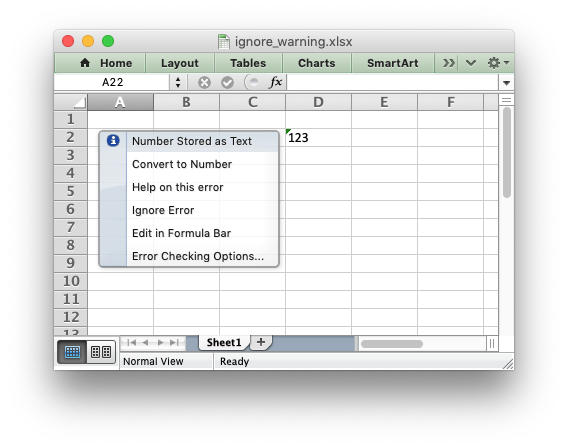Sometimes these warnings are useful indicators that there is an issue in the spreadsheet but sometimes it is preferable to turn them off. Warnings can be turned off at the Excel level for all workbooks and worksheets by using the using “Excel options -> Formulas -> Error checking rules”. Alternatively you can turn them off for individual cells in a worksheet, or ranges of cells, using the `ignore_errors()` method with a dict of options and ranges like this:

```worksheet.ignore_errors({'number_stored_as_text': 'A1:H50'})

# Or for more than one option:
worksheet.ignore_errors({'number_stored_as_text': 'A1:H50',
'eval_error':            'A1:H50'})
```

The range can be a single cell, a range of cells, or multiple cells and ranges separated by spaces:

```# Single cell.
worksheet.ignore_errors({'eval_error': 'C6'})

# Or a single range:
worksheet.ignore_errors({'eval_error': 'C6:G8'})

# Or multiple cells and ranges:
worksheet.ignore_errors({'eval_error': 'C6 E6 G1:G20 J2:J6'})
```

Note: calling `ignore_errors()` multiple times will overwrite the previous settings.

You can turn off warnings for an entire column by specifying the range from the first cell in the column to the last cell in the column:

```worksheet.ignore_errors({'number_stored_as_text': 'A1:A1048576'})
```

Or for the entire worksheet by specifying the range from the first cell in the worksheet to the last cell in the worksheet:

```worksheet.ignore_errors({'number_stored_as_text': 'A1:XFD1048576'})
```

The worksheet errors/warnings that can be ignored are:

• `number_stored_as_text`: Turn off errors/warnings for numbers stores as text.
• `eval_error`: Turn off errors/warnings for formula errors (such as divide by zero).
• `formula_differs`: Turn off errors/warnings for formulas that differ from surrounding formulas.
• `formula_range`: Turn off errors/warnings for formulas that omit cells in a range.
• `formula_unlocked`: Turn off errors/warnings for unlocked cells that contain formulas.
• `empty_cell_reference`: Turn off errors/warnings for formulas that refer to empty cells.
• `list_data_validation`: Turn off errors/warnings for cells in a table that do not comply with applicable data validation rules.
• `calculated_column`: Turn off errors/warnings for cell formulas that differ from the column formula.
• `two_digit_text_year`: Turn off errors/warnings for formulas that contain a two digit text representation of a year.